• 机器学习笔记——受限玻尔兹曼机简介
2021-05-25 20:17:46

整理自：http://blog.echen.me/2011/07/18/introduction-to-restricted-boltzmann-machines/

# 0x01 intro

假设我们让一群用户给一堆电影进行评分（满分100）。在经典的因子分析中，我们可以试着用一堆潜在因素来解释用户和因子的关系。比如，像《星球大战》和《指环王》这样的电影和科幻高度相关，而《Wall-E》和《玩具总动员》的用户可能与潜在的“皮克斯”因素有很强的联系。

限制玻尔兹曼机可以理解为是因子分析的二进制版本，比如一个电影有没有科幻/搞笑/动作元素啥的。用户的输入只是它喜不喜欢啥电影，然后输出告诉我们这个用户可能是二次元/科幻/blablabla，相当于是用特定电影的喜好与否给用户画像。

技术上理解，受限玻尔兹曼机是随机神经网络。它由两层东西构成：

• 一层可视化层（对电影的评价）
• 一层隐藏层（这个用户喜欢啥风格）

当然，隐藏层和可见层的每个神经元都有偏置项。

一个典型的例子如下所示：（这里，右边的那个黑点子是偏置项）

# 0x02 工作原理

和神经网络的全连接类似，受限玻尔兹曼机的两层之间也是全连接的，但是层内的神经元是不连接的。下面，我们来介绍一下受限玻尔兹曼机的使用原理。

以上图所示，我们输入用户对六个电影的打分结果（0-100分）。然后，我以分数百分比为概率对输入进行0-1取样，比如用户给哈利波特打90分，咱们就以90%的可能性把这个点取成1，10%的可能性取成0。

对每个输入点取过样之后，我们和神经网络前向传播一样，计算一个线性组合 ∑ w i j x j + b i \sum w_{ij} x_j + b_i ，这里 x j x_j 就是我们的每个输入， b i b_i 是输出节点的bias。

得到的线性组合扔到sigmoid函数里，得到一个0-1之间的连续值。我们再以这个值为概率做一手前面说的0-1取样。

（概括一手，如果 w i j w_{ij} 是正的，那么前后两个神经元就想共享同一个状态，比如《流浪地球》和科幻之间的 w i j w_{ij} 肯定得是个超大的正数）

# 0x03 学习权重

可以发现，这里对网络的输出起作用的就是权重和偏置bias了。假设我们的测试用例是1行6列的二进制向量。在每个训练epoch，做下面的操作：

1. 把测试用例扔到输入层里。
2. 正向传播，并且以sigmoid函数值对每个隐藏层神经元做取样。
3. 计算一下 e i j e_{ij} ，其定义为输入层第 i i 个单元和输出层第 j j 个单元是否都被激活（即值为1）。都被激活则为1，否则为0.
4. 下面，我们把3中隐藏层算出的值当成隐藏层的输入，根据这个输入向可见层做一个传播，做法仍然是线性组合之后再套一个sigmoid函数，只不过和2中的方向是反的。
5. 再计算一个新的 f i j f_{ij} ，定义和3中类似。
6. 更新： w i j = w i j + γ ( e i j − f i j ) w_{ij} = w_{ij} + \gamma (e_{ij} - f_{ij})

这个步骤叫对比散度（contrastive divergence），但是本质上还是近似梯度下降。

# 0x04 why?

这里就放一个直观的理解：

• e i j e_{ij} 表示的是我们希望网络学到的东西，学到哪些东西是要激活的。
• 在反向传播的阶段，RBM只是根据咱们正向传播的假设来生成可见层的值。 f i j f_{ij} 就表示没有固定输入的情况下测量网络本身生成的训练样本。

一个特殊的情况是，假设根据隐藏层构建的东西和原本输入的东西严格相等，这个时候网络就不用更新了，观察e和f，则有e和f严格相等。

更多相关内容
• 深度学习 之 玻尔兹曼机 1.玻尔兹曼 BM 首先BM中的神经元都是二值神经元,也就是要么是0要么是1 如何理解上图中的BM与一般神经网络的区别的呢? 我们可以在图中看到,它是个无向完全图 也就是说,每个节点与节点之间都...

# 深度学习 之 玻尔兹曼机

## 1.玻尔兹曼 BM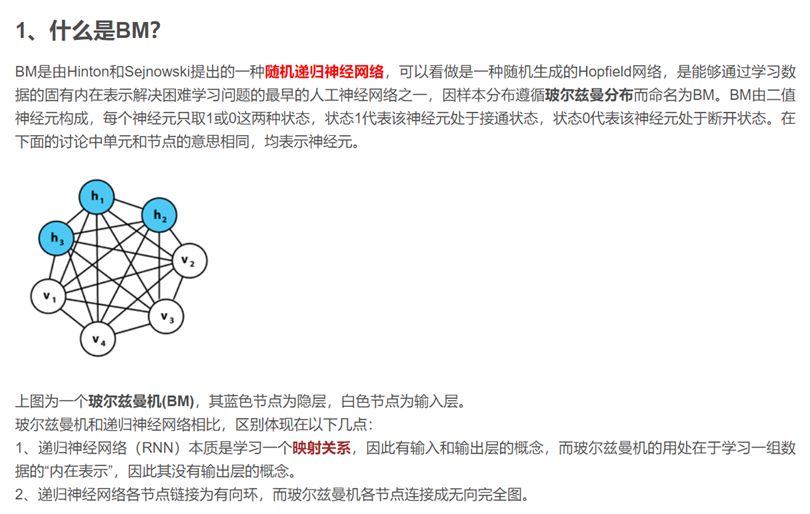首先BM中的神经元都是二值神经元,也就是要么是0要么是1
如何理解上图中的BM与一般神经网络的区别的呢?

• 我们可以在图中看到,它是个无向完全图
• 也就是说,每个节点与节点之间都相连, 并且 节点与节点之间是双向的关系

这与一般神经网络不同,正常的神经网络中都分输入层,隐藏层,输出层, 每次训练都是按着一定的顺序往后推进的*

• 另外,在BM中,我们无法找到哪个是输入口,哪个是输出口,因为BM的作用是仅仅在自己内部训练,学习一组数据的内在表示

## 2.限制玻尔兹曼 RBM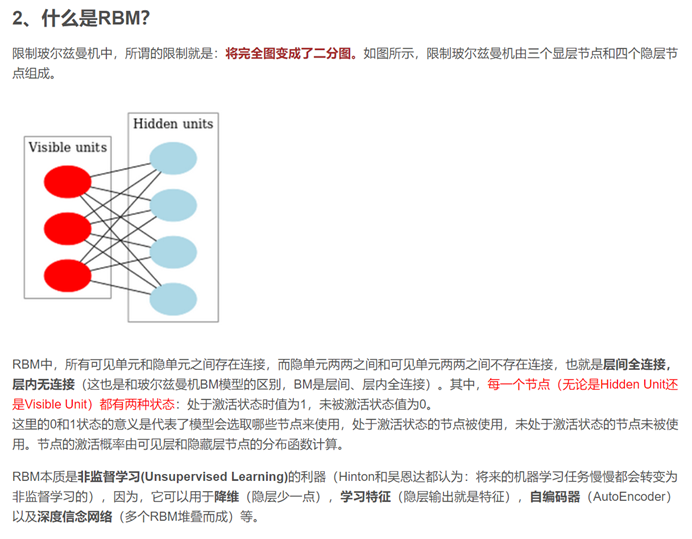**在图中我们可以看到 RBM相比于BM去掉了一些神经元直接的连接, 比如红色与红色之间 蓝色与蓝色之间, 不过同样的,神经元与神经元之间的连接是无向的 **
他的本质是无监督学习,如上图中所述,他是很多方法的基础,比如降维,自编码器,学习特征等等
下图给出了RBM的样子, 它只有输入层和隐藏层,并没有输出层的概念,而我们刚刚学习来看,他是层间全连接,层内无连接的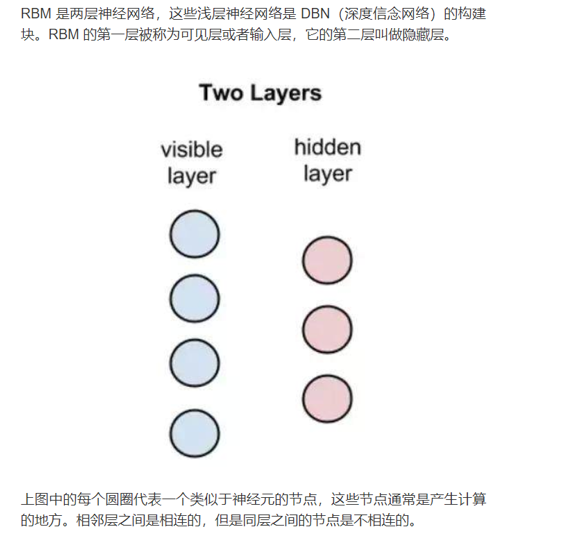我们来看看RBM的训练过程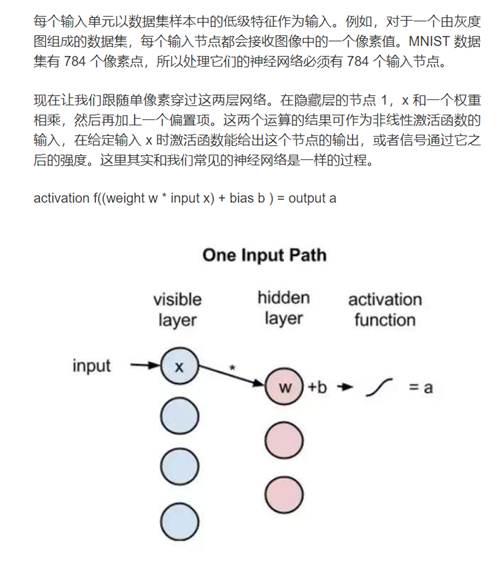前向传播的过程其实和常见的神经网络是一样的,输入x
经过一层隐藏层之后得到 a = s i g m o i d ( w ∗ x + b ) a = sigmoid(w*x+b)
如果有多层隐藏层的话,如下图所说的,这里的RBM节点就起着自编码器的作用,也就是起到了降维的作用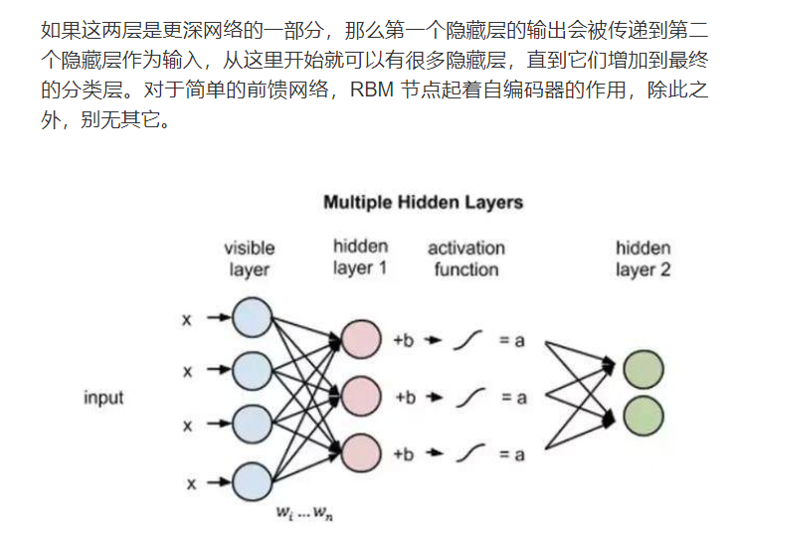重建的过程简单的说就是倒着训练,我们先看看上面那张图 结果是 a = s i g m o i d ( w ∗ x + b ) a =sigmoid(w*x+b)
现在在重建的过程中,我们的公式变成了 r = s i g m o i d ( v + w ∗ a ) r = sigmoid(v + w*a)
而RBM的目标就是在若干次训练后,让我们低纬度的a能跟r近似,也就是说在降维的同时保留了大部分原来的信息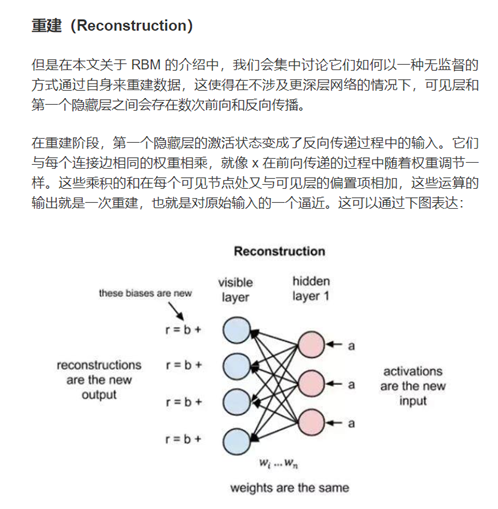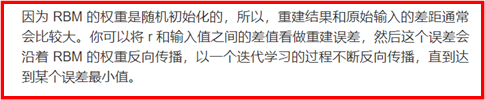前向传播预测的是 a的输出概率 已知 x 和 w的情况下
反向传播预测的是 x(也可以说r)的概率 ,在已知a 和 w的情况下
这就和刚刚的式子对应上了,这不过这里预测的是概率
而我们的目标是使得这两个p越接近越好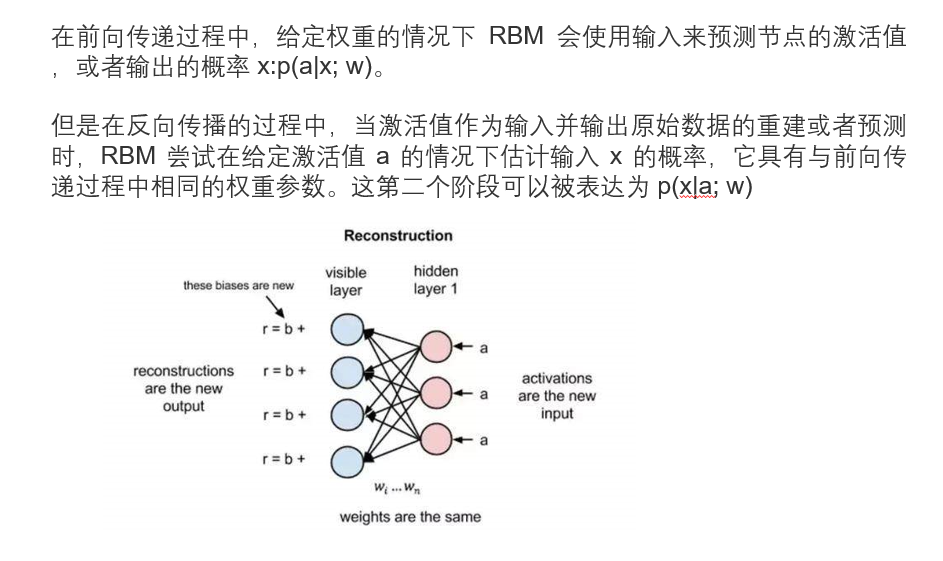如下图所说,把两个概率结合起来写成 p ( x , a ) p(x,a) 也就是输入x与激活值a的联合概率分布
如下图所说: 这里的重建被称为生成学习, 与我们之前所学的分类器是不一样的,下面我就举一个简单的例子
对于我们之前所学习的分类器, 比如我们给他一张猪的照片和一张戴眼镜的人的照片, 分类器返回的是 标签:(猪,人),而生成学习返回的是数据,他会返回一个戴眼镜的猪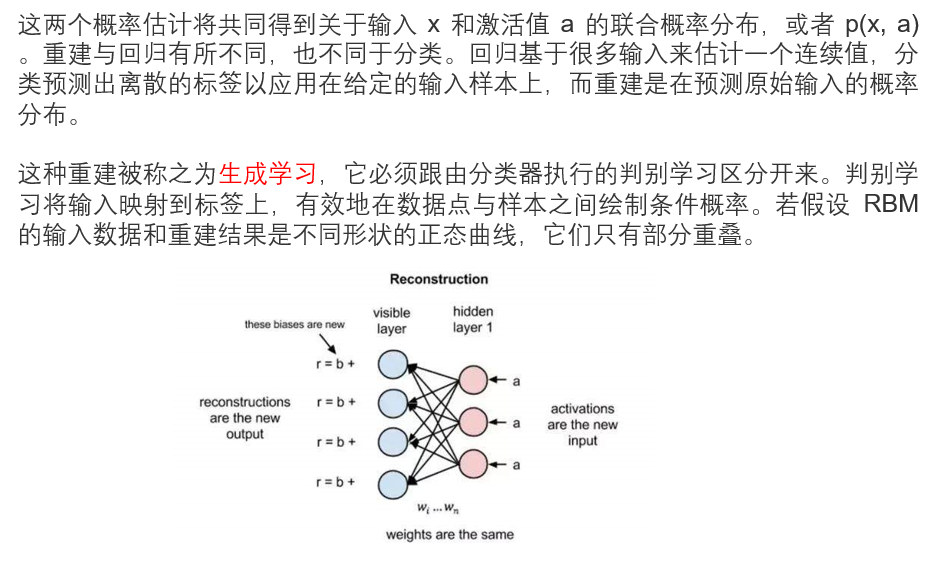而RBM的重建的目标就是是的输入数据和重建结果的曲线重合部分越多越好,简单画个图
比如黑色的正态曲线是原来的输入x的分布,而重建的结果是红线,他与原来代表x的黑线重叠部分越多越好,也就是说,我们通过重接得到的结果,越接近原来的值越好,这点我在之前已经提到过了,这不过现在我们把这个概念转化成了概率分布的重叠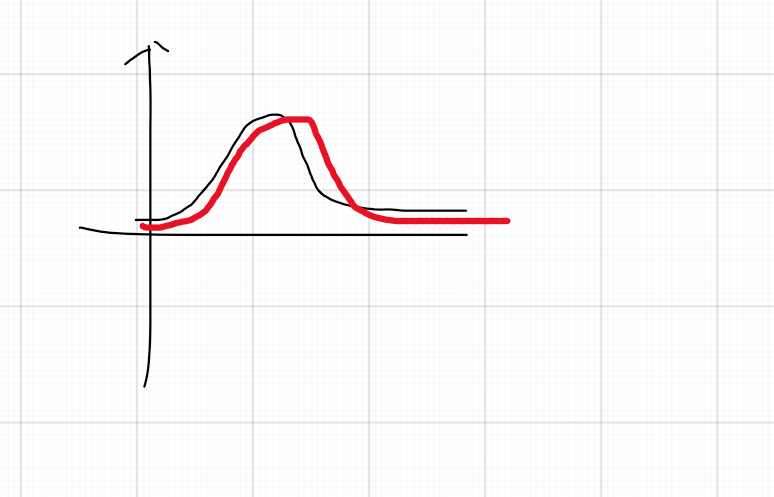下图的第一个函数表示的是一个RBM的能量 , 因为是双向连接的 所以 后面的两个公式 就是从头到尾与从尾到头的计算公式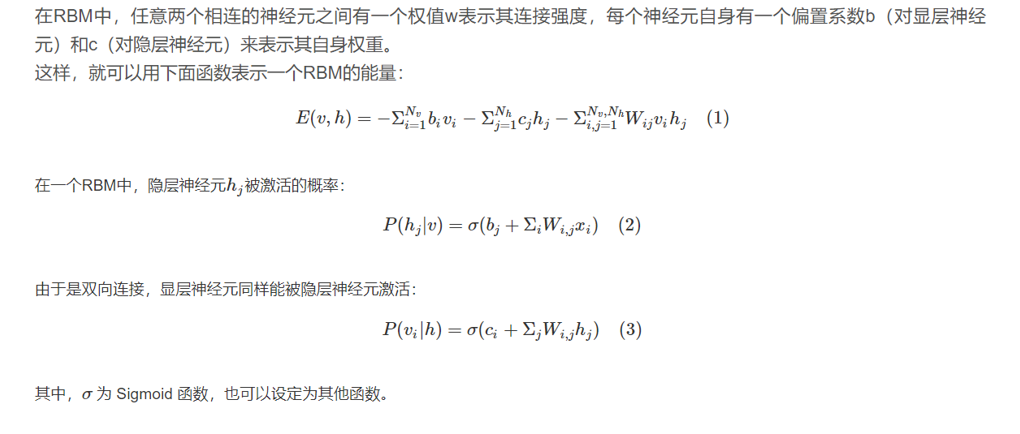这个能量函数的意思就是，每个可视节点和隐藏节点之间的连接结构都有一个能量，通俗来说就是可视节点的每一组取值和隐藏节点的每一组取值都有一个能 量，如果可视节点的一组取值（也就是一个训练样本的值）为(1,0,1,0,1,0)，隐藏节点的一组取值（也就是这个训练样本编码后的值）为 (1,0,1)，然后分别代入上面的公式，就能得到这个连接结构之间的能量。
能量函数的意义是有一个解释的，叫做专家乘积系统（POE，product of expert），这个理论也是hinton发明的，他把每个隐藏节点看做一个“专家”，每个“专家”都能对可视节点的状态分布产生影响，可能单个“专家” 对可视节点的状态分布不够强，但是所有的“专家”的观察结果连乘起来就够强了。

## KL散度

之前已经说了,我们的目的是重建得到的目标分布跟输入数据的分布曲线接近, 也就是下图中的p(x)与q(x) 下面的右图是它们差的积分, 理解起来就是每一点p(x)与q(x)的差值所组成的函数,然后对它求积分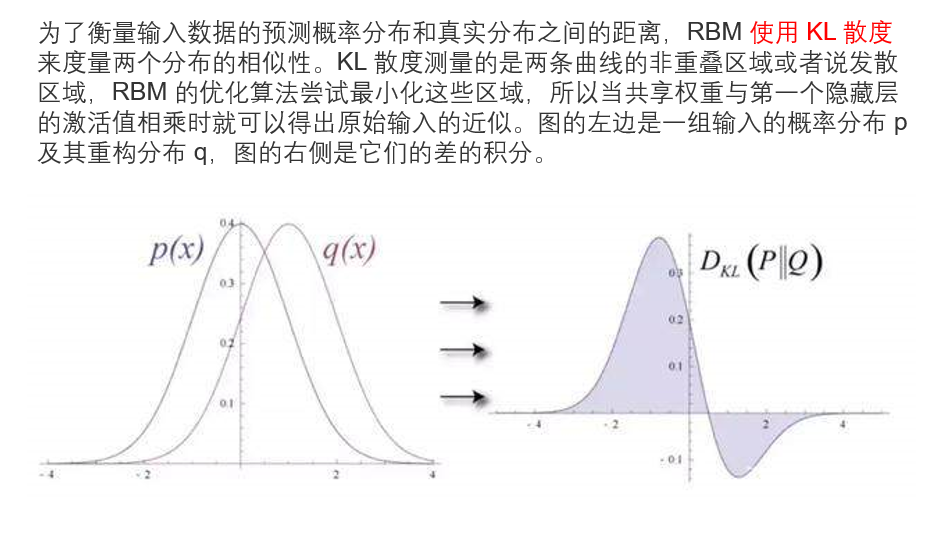KL散度测量的就是两条曲线不重叠的部分, 而我们的目的就是使得KL散度越小越好
如下图说的,学习的过程就像两个概率分布在逐步重合,也就是两个曲线越来越接近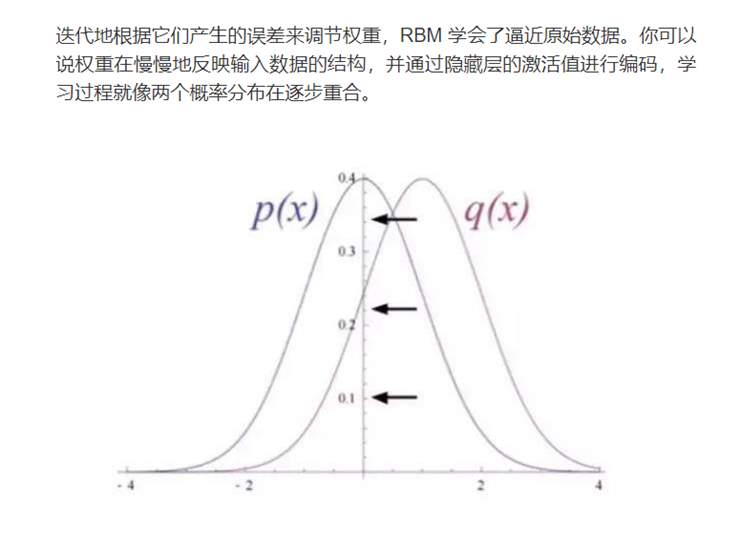对于KL散度的通俗理解可以看这篇文章, 举了太空虫子为案例,蛮有趣的
多层受限玻尔兹曼机也很好理解.就是一层一层的学习,知道神经网络的话就十分好理解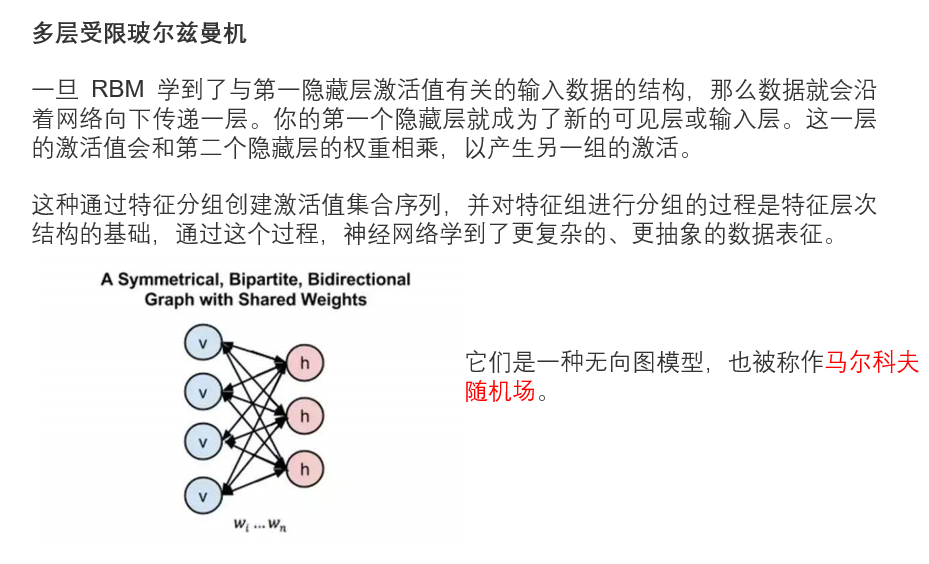之前将了玻尔兹曼机的实现原来,下面更加细节的介绍一下,中间迭代的过程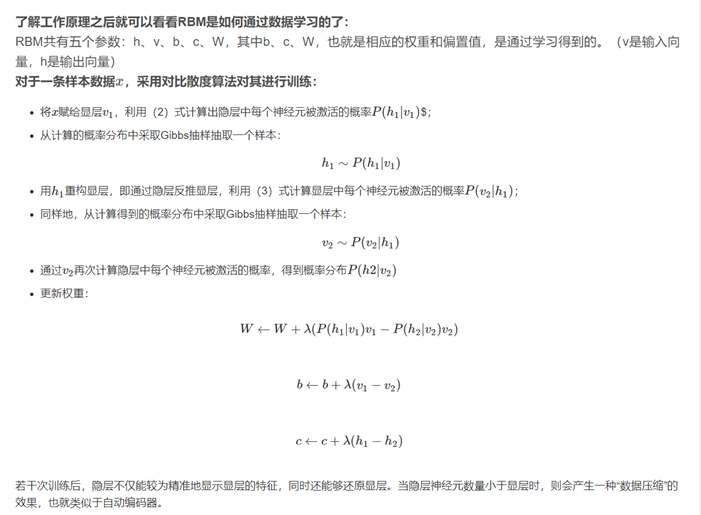在我们之前回归训练的时候 损失函数 loss是这样的
l o s s = 1 2 ∑ ( y ^ − y ) 2 loss = \frac{1}{2}\sum(\hat{y}-y)^2
而在这里我们的损失函数是这样的 ,具体可以查看KL散度的内容,用到了信息熵的概念
l o s s = ∑ p ( x ) ∗ p ( x ) q ( x ) loss = \sum{p(x)*\frac{p(x)}{q(x)}}
我们的目的就是让损失最小
在之前我们使用的方法是梯度下降法,这里的方法跟梯度下降类似,只不过因为这个方法提出的时间可能比较早,所以在梯度下降的过程中没有计算导数
公式如下如 , 我们可以看到,它是直接进行做差,然后乘一个学习率,而我们之前学到的是学习率乘损失的导数,这里就这点不太一样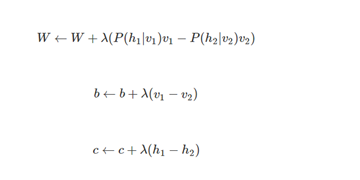在经过一轮轮的训练之后,我们就完成了效果,及训练完的参数可以使得重建之后的结果与输入数据的分布曲线损失最小
注: 我们现在使用的压缩软件其实内部也可以用神经网络来实现 ,比如1个G的文件压缩成500M,解压之后还是原来的内容

展开全文深度学习
•深度置信网络
• Boltzmann Machines A Beginner’s Guide to Restricted Boltzmann Machines (RBMs) 深度学习基础：Boltzmann Machines 受限玻尔兹曼机基础教程 受限玻尔兹曼机（RBM）学习笔记 基于能量的模型和波尔兹曼机 深度学习...

## Boltzmann Machines起源

在上一篇博客Hopfield神经网络学习(HNN)中，我们提到Hopefield网络容易发生串扰或陷入局部最优解，导致网络不能正确的识别模式，Boltzmann Machines通过让每个单元按照一定的概率分布发生状态变化可以解决这个问题。

## Boltzmann Machines的结构

Boltzmann Machines保持了Hopefield的假设：

• 权重对称
• 自身无连接
• 二值输出

输出单元 i ​ i​ 有一定的概率改变自身的值，首先需要计算它的总输入 z i ​ z_i​ :
(1) z i = b i + ∑ j s j w i j ​ z_i=b_i+\sum_js_jw_{ij}\tag{1}​
上式中 w i j ​ w_{ij}​ 是连接单元 i ​ i​ j ​ j​ 的权重，然后单元 i ​ i​ 会按照下面的概率更新自身：
(2) p r o b ( s i = 1 ) = 1 1 + e − z i ​ prob(s_i=1)=\frac{1}{1+e^{-z_i}}\tag{2}​

当所有的神经元都不再更新的时候，网络达到了一个平稳状态（参考马尔科夫链的平稳分布），即Boltzmann Distribution，在这个分布中，所有状态 v ​ v​ 的概率取决于状态空间中该状态的能量。
(3) P ( v ) = e − E ( v ) ∑ u e − E ( u ) ​ P(v)=\frac{e^{-E(v)}}{\sum_ue^{-E(u)}}\tag{3}​
分母是所有状态的能量之和，分子是当前状态的能量，即每个状态的能量的归一化比例，一个状态的能量越小其出现概率越高。

我们在Hopefield网络中对每一个状态的能量已经有了定义：
(4) E ( v ) = − ∑ i s i v b i − ∑ i &lt; j s i v s j v w i j E(v)=-\sum_is_i^vb_i-\sum_{i&lt;j}s_i^vs_j^vw_{ij}\tag{4}
其中 s i v s_i^v 是状态v第i个神经元的二值状态。

玻尔兹曼机可以用来解决两类问题。一类是搜索问题。当给定变量之间的连接权重，需要找到一组二值向量，使得整个网络的能量最低。另一类是学习问题。当给一组定部分变量的观测值时，计算一组最优的权重。

## Boltzmann Machines的搜索问题

在玻尔兹曼机中，配分函数Z 通常难以计算，因此，联合概率分布p(x)一般通过MCMC方法来近似，生成一组服从p(x)分布的样本。本节介绍基于吉布斯采样的样本生成方法。

吉布斯采样需要计算每个变量X i 的全条件概率KaTeX parse error: Expected '}', got '\i' at position 10: p(x_i|x_{\̲i̲})，其中KaTeX parse error: Expected 'EOF', got '\i' at position 2: x\̲i̲表示除变量 x i x_i 外其它变量的取值。

(5) p r o b ( s i = 1 ) = 1 1 + e b i + ∑ j s j w i j prob(s_i=1)=\frac{1}{1+e^{b_i+\sum_js_jw_{ij}}}\tag{5}

不同于Hopefield网络，Boltzmann Machines加入了对神经元更新自身时的概率限制，可以帮助网络跳出局部最小点，从而能够得到更优的解。具体来讲就是：我们在讲Hopefield网络的时候谈到，为了让每次状态变化的时候能量变换量都小于0，我们设计了能量变化量的计算公式，但是如果我们以一定的概率舍弃神经单元的更新，那么这个假设便不成立了，也就是说能量可能会上升，而不是一直下降，这就给了网络一个跳出局部极小点的机会。

这个思想类似于模拟退火的思想，模拟退火是一种寻找全局最优的近似方法，其名字来自冶金学的专有名词“退火”，即将材料加热后再以一定的速度退火冷却，可以减少晶格中的缺陷。固体中的内部粒子会停留在使内能有局部最小值的位置，加热时能量变大，粒子会变得无序并随机移动。退火冷却时速度较慢，使得粒子在每个温度都达到平衡态。最后在常温时，粒子以很大的概率达到内能比原先更低的位置。可以证明，模拟退火算法所得解依概率收敛到全局最优解

## Boltzmann Machines的学习问题

### 不含隐藏层的学习

给定某一状态空间下的训练集（数据），学习出权重矩阵、偏置矩阵，使得这些训练样本的对数似然函数尽可能的高，即EBM(Energy Based Model)模型中的学习问题。

通过对Eq.3和Eq.4求导我们可以得到：
∂ E ( v ) ∂ w i j = − s i v s j v ​ \frac{\partial E(v)}{\partial w_{ij}}=-s_i^vs_j^v​
∂ l o g P ( v ) ∂ w i j = &lt; s i s j &gt; d a t a − &lt; s i s j &gt; m o d e l ​ \frac{\partial logP(v)}{\partial w_{ij}}=&lt;s_is_j&gt;_{data}-&lt;s_is_j&gt;_{model}​

有了目标函数，接下来我们只需要应用梯度上升算法，在训练集上进行训练，最大化所有样本的对数概率，就能够得到一组理想的参数。

如果训练样本中对每一个神经元都赋予了一个0-1值，那么这个问题就是一个凸优化问题，存在且只存在一个全局最优解，但是往往训练样本的空间的维度小于Boltzmann Machines的状态空间的维度，因此存在多个局部最小值。

### 包含隐藏层的学习

当Boltzmann Machines网络中既包含可被观察到的可见神经元，又包含不可见神经元的时候，网络可以表达更加复杂的模式，就像上面我们说的，样本数据的维度往往小于Boltzmann Machines状态空间的维度，但是我们又无法直接扩充训练集的维度，因此我们在网络中加入隐藏单元，这样网络就可以在隐藏单元和可见单元之间建立联系，而不仅仅是在可见单元之间建立联系，而这些不可见单元的值并没有在训练样本中指定，这使得我们能够捕获更加高阶的数据分布。

加入隐藏单元之后，我们的学习算法并不需要做出改变，但是隐藏单元的值需要一直更新直到达到稳定分布。

### 学习速度

包含多层隐藏单元和可见单元的Boltzmann Machines的学习是非常慢的，因为网络越大，达到稳定分布所需要的时间就越长，尤其是当权重矩阵的值较大的时候，最终达到的稳定分布很有可能是高维多峰的。我们可以通过限制连接、简化学习算法或者依次只学习一个隐藏层来缓解这个问题。

## 不同类型的Boltzmann Machines

### High-Order Boltzmann Machines

Boltzmann Machines的动态随机算法和学习算法可以使用更加复杂的能量函数，比如能量函数中的第二项可以替换为 s i s j s k w i j k s_is_js_kw_{ijk} ，然后总输入 z z 也需要变成 z i = b i + ∑ j &lt; k s j s k w i j k z_i = b_i + \sum_{j&lt;k}s_js_kw_{ijk}

### Conditional Boltzmann Machines

Boltzmann Machines 建模了数据的分布，我们可以将其扩展一下用来对条件分布进行建模。当我们计算 &lt; s i s j &gt; d a t a &lt;s_is_j&gt;_{data} 的时候，计算了可见单元的值，但是没有计算隐藏单元的值，如果我们取出一部分可见单元做为input，并从中抽样作为样本，那么其余的可见单元就是output，我们就得到了条件概率 P ( o u t p u t ∣ i n p u t ) P(output|input) 的建模，学习算法仍然不变。

没看懂

### Non-Binary Units

Boltzmann Machines的神经单元默认都是二值的，只有0和1两个取值，我们可以将其泛化到softmax单元、高斯单元、泊松单元或者其他任何属于指数簇的单元，使其拥有更多的取值。这些单元的特性是其参数的变化对对数概率的影响是线性的，因此我们不需要修改学习算法。

## Restricted Boltzmann Machines

当Boltzmann Machines 包含很多可见单元层和隐藏单元层的时候会导致网络的训练特别困难，因此在其上加一些限制，使得层内单元之间没有连接，如下图所示：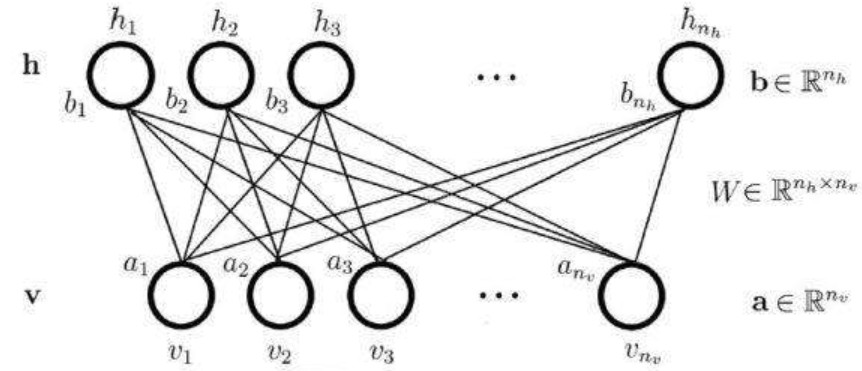## 参考文献

Boltzmann Machines

A Beginner’s Guide to Restricted Boltzmann Machines (RBMs)

深度学习基础：Boltzmann Machines

受限玻尔兹曼机基础教程

受限玻尔兹曼机（RBM）学习笔记

基于能量的模型和波尔兹曼机

深度学习读书笔记之RBM(限制波尔兹曼机)

一起读懂传说中的经典：受限玻尔兹曼机

展开全文• 受限玻尔兹曼机和深度置信网络 引言 梯度弥散一直是困扰着深度神经网络的发展，那么如何解决梯度弥散问题呢？多伦多大学的Geoff Hinton提出了设想：受限玻尔兹曼机（Restricted Boltzmann Machines, RBM），即...

### 从玻尔兹曼机到深度置信网络

本文仍处于草稿阶段，请慎重观看

#### 引言

• 受限玻尔兹曼机（Restricted Boltzmann Machines, RBM）最初是在1986年由Paul Smolensky发明，在Geoffrey Hinton和合作者在2005年左右为其发明了快速学习的算法之后，RBM得以进一步发展。RBM可用于可用于降维、分类、回归、协同过滤、特征学习和主题建模。根据任务，RBM可用于监督学习或无监督学习。

• RBM也可用于深度学习网络。具体而言，深度置信网络（Deep Belief Networks）可以通过“堆叠”RBM并可选地通过梯度下降和反向传播来微调网络的参数。

• 本文将从玻尔兹曼机的结构开始介绍，其后引申至受限玻尔兹曼机，最后介绍由受限玻尔兹曼机堆叠而成的深度置信网络。

#### 1. 玻尔兹曼机

玻尔兹曼机(Boltzmann Machine，BM)是一个为整个网络定义“能量”的单元网络。BM的节点产生二元结果（0或1），一个玻尔兹曼机可以表示为带权重的无向图：由上图可以看出BM的结构为层间、层内全连接。由于每个节点是二值的，所以一共有 2n 2 n $2^n$个状态，对于一个节点 xi x i $x_i$，其值为1 的时候表示这个节点是’on’，其值为0的时候表示这个节点是’off’。

与Hopfield网络不同，玻尔兹曼机节点是随机的。而BM的能量形式与Hopfield网络的形式相同：

E=(i<jwijsisj+iθisi) E = − ( ∑ i < j w i j s i s j + ∑ i θ i s i )

其中， wij w i j $w_{ij}$为节点 i i $i$ j j $j$ 之间的连接权重； si s i $s_i$为节点 i i $i$ 的状态，其值为0或1；${\theta }_{i}$$\theta_i$为节点 i i $i$的偏置。

##### 1. 1 玻尔兹曼机节点状态概率

BM的全局能量差值由每个节点的状态差值产生，由下式给出：

$\mathrm{\Delta }{E}_{i}={E}_{i=0}-{E}_{i=1}$

#### 2. 受限玻尔兹曼机

无连接约束(层间层内全连接)的波尔兹曼机被证明在机器学习实际问题中效果不佳，但是如果节点的连接受到适当限制，则可以使学习效果足够有效以用于解决实际问题。
受限玻尔兹曼机是玻尔兹曼机的一种变体，区别于玻尔兹曼机，受限玻尔兹曼机可见节点和隐含节点之间存在连接，而隐含节点两两之间以及可见节点两两之间不存在连接，也就是层间全连接，层内无连接。

RBM可以表示成一个二分图模型，所有可见层节点和隐藏层节点都有两种状态：处于激活状态时值为1，未被激活状态值为0。这里的0和1状态的意义是代表了模型会选取哪些节点来使用，处于激活状态的节点被使用，未处于激活状态的节点未被使用。节点的激活概率由可见层和隐藏层节点的分布函数计算。

下面给出RBM模型的数学化定义：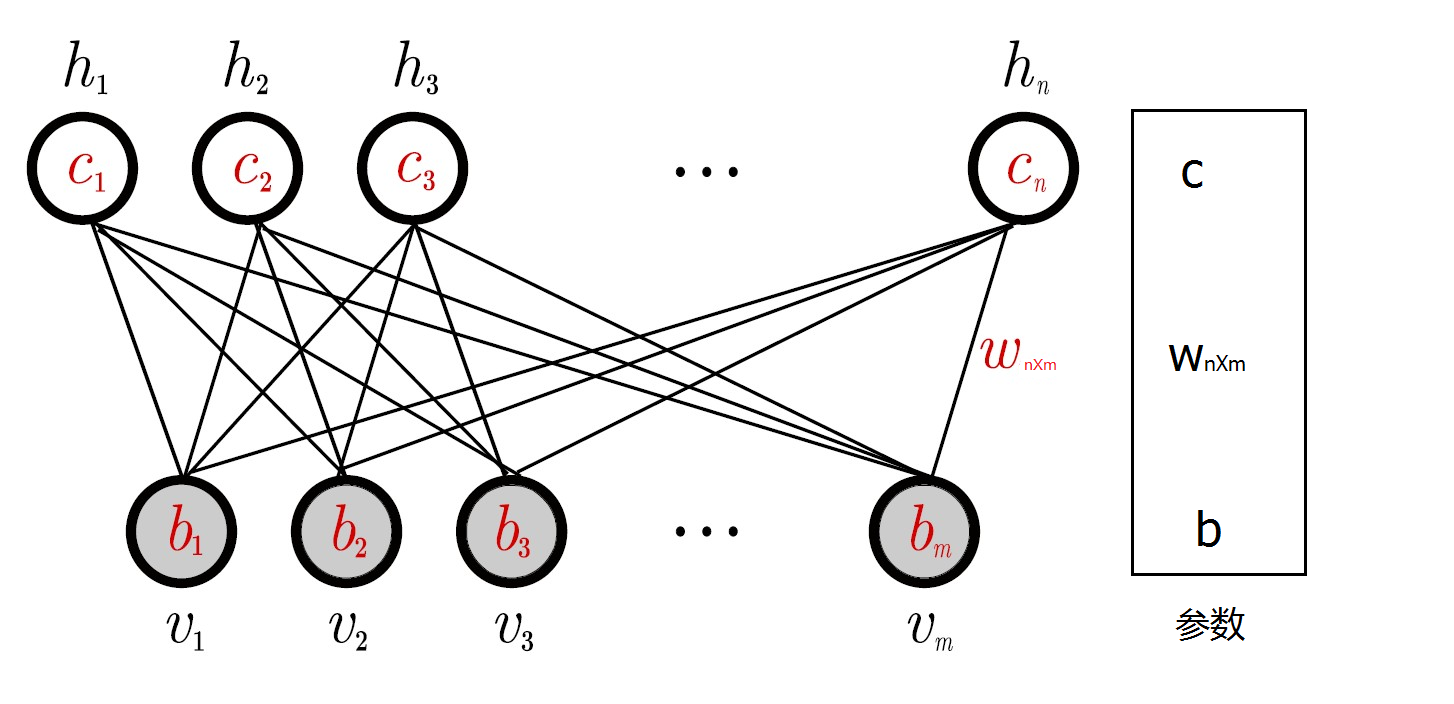如图1所示，一个RBM包含一个由随机的隐含节点构成的隐藏层(一般是伯努利分布)和一个由随机的可见节点构成的可见层(一般是伯努利分布或高斯分布)。

RBM有如下性质：
当给定可见层神经元的状态时，各隐藏层神经元的之间是否激活是条件独立的；反之也同样成立。

##### 2.1 受限玻尔兹曼机参数学习

RBM中， v v $v$表示所有可见单元，$h$$h$表示所有隐单元。要想确定该模型，只要能够得到模型三个参数 θ={W,A,B} θ = { W , A , B } $\theta=\{W,A,B\}$即可。分别是权重矩阵 W W $W$，可见层单元偏置$A$$A$，隐藏层单元偏置 B B $B$
假设一个RBM有$n$$n$个可见单元和 m m $m$个隐单元，用${v}_{i}$$v_i$表示第 i i $i$个可见单元，${h}_{i}$$h_i$表示第 j j $j$个隐单元，它的参数形式为：
$W=\left\{{w}_{i,j}\in {R}^{n×m}\right\}$$W=\{w_{i,j}\in R^{n\times m}\}$ ，其中 Wi,j W i , j $W_{i,j}$表示第 i i $i$个可见单元和第$j$$j$个隐单元之间的权值
A={aiRm} A = { a i ∈ R m } $A=\{a_i\in R^m\}$ ，其中 ai a i $a_i$表示第 i i $i$个可见单元的偏置阈值
$B=\left\{{b}_{j}\in {R}^{n}\right\}$$B=\{b_j\in R^n\}$ ，其中 bj b j $b_j$表示第 j j $j$个可见单元的偏置阈值
对于一组给定状态下的$\left(v,h\right)$$(v, h)$值，假设可见层单元和隐藏层单元均服从伯努利分布，RBM的能量公式是：

E(v,h|θ)=i=1naivij=1mbjhji=1nj=1mviWijhj E ( v , h | θ ) = − ∑ i = 1 n a i v i − ∑ j = 1 m b j h j − ∑ i = 1 n ∑ j = 1 m v i W i j h j
其中， θ={Wij,ai,bj} θ = { W i j , a i , b j } $\theta=\{W_{ij},a_i,b_j\}$是RBM模型的参数，能量函数表示在每一个可见节点的取值和每一个隐藏层节点的取值之间都存在一个能量值。

对该能量函数指数化和正则化后可以得到可见层节点集合和隐藏层节点集合分别处于某一种状态下 (v,h) ( v , h ) $(v,h)$联合概率分布公式：

P(v,h|θ)=eE(v,h|θ)Z(θ) P ( v , h | θ ) = e − E ( v , h | θ ) Z ( θ )
Z(θ)=v,heE(v,h|θ) Z ( θ ) = ∑ v , h e − E ( v , h | θ )
其中， Z(θ) Z ( θ ) $Z(\theta)$为归一化因子或配分函数（partition function），表示对可见层和隐藏层节点集合的所有可能状态的能量指数求和。

对于参数的求解往往采用似然函数求导的方法。已知联合概率分布 P(v,h|θ) P ( v , h | θ ) $P(v,h|\theta)$，通过对隐藏层节点集合的所有状态求和，可以得到可见层节点集合的边缘分布 P(v|θ) P ( v | θ ) $P(v|\theta)$

P(v|θ)=1Z(θ)heE(v,h|θ) P ( v | θ ) = 1 Z ( θ ) ∑ h e − E ( v , h | θ )
边缘分布表示的是可见层节点集合处于某一种状态分布下的概率。

由于RBM模型的特殊的层间连接、层内无连接的结构，它具有以下重要性质：
1）在给定可见单元的状态时，各隐藏层单元的激活状态之间是条件独立的。此时，第 j j $j$个隐单元的激活概率为：

$P\left({h}_{j}=1|v\right)=\sigma \left({b}_{j}+\sum _{i}{v}_{i}{W}_{ij}\right)$
2）相应的，当给定隐单元的状态时，可见单元的激活概率同样是条件独立的：

P(vi=1|h)=σ(ai+jWijhj) P ( v i = 1 | h ) = σ ( a i + ∑ j W i j h j )
其中， σ(x)=11+exp(x) σ ( x ) = 1 1 + e x p ( − x ) $\sigma(x)=\frac{1}{1+exp(-x)}$是sigmoid函数，其函数曲线如下图所示：采用该函数用作每一层节点的激活概率公式的原因是：sigmoid函数的定义域是 (,+) ( − ∞ , + ∞ ) $(-\infty,+\infty)$，值域处于 (0,1) ( 0 , 1 ) $(0,1)$之间。也就是说，无论模型的可见层输入节点数据处于一个多大的范围内，都可以通过sigmoid函数求得它相应的函数值，并且该函数值被压缩于0到1之间，即节点的激活概率值。

##### 2.2 受限玻尔兹曼机模型参数求解

确定RBM模型需要求解模型的三个参数 θ={Wij,ai,bj} θ = { W i j , a i , b j } $\theta=\{W_{ij},a_i,b_j\}$，下面围绕参数的求解进行分析。

参数求解使用对数似然函数对参数求导。
P(v|θ)=1Z(θ)heE(v,h|θ) P ( v | θ ) = 1 Z ( θ ) ∑ h e − E ( v , h | θ ) $P(v|\theta)=\frac{1}{Z(\theta)}\sum_he^{-E({v,h|\theta})}$可知，能量 E E $E$和概率$P$$P$成反比，通过最大化 P P $P$来最小化$E$$E$。最大化似然函数常用方法是梯度上升法，梯度上升法是指按照以下公式对参数进行修改：

θ=θ+μlnP(v)θ θ = θ + μ ∂ l n P ( v ) ∂ θ
通过求 lnP(v) l n P ( v ) $lnP(v)$关于 θ θ $\theta$的导数，即 Δθ Δ θ $\Delta\theta$，然后对原 θ θ $\theta$值进行修改。如此迭代使似然函数 P P $P$最大，从而使能量$E$$E$最小。
对数似然函数的格式： lnP(vs) l n P ( v s ) $lnP(v^s)$ vs v s $v^s$表示模型的输入数据，此处先对单个样本进行分析，即 vs v s $v^s$为数据集中第 s s $s$个样本。
然后对$\left\{{W}_{ij},{a}_{i},{b}_{j}\right\}$$\{W_{ij},a_i,b_j\}$里的参数分别进行求导，详细的推导过程就不写了：
lnP(vs)wi,j=P(hi=1|vs)vsjvP(v)P(hi=1|v)vj ∂ l n P ( v s ) ∂ w i , j = P ( h i = 1 | v s ) v j s − ∑ v P ( v ) P ( h i = 1 | v ) v j

lnP(vs)ai=vsivP(v)vi ∂ l n P ( v s ) ∂ a i = v i s − ∑ v P ( v ) v i

lnP(vs)bi=P(hi=1|vs)vP(v)P(hi=1|v) ∂ l n P ( v s ) ∂ b i = P ( h i = 1 | v s ) − ∑ v P ( v ) P ( h i = 1 | v )
由于上面三式的第二项中都含有 P(v) P ( v ) $P(v)$ P(v) P ( v ) $P(v)$中仍然含有参数，所以它是式中求不出来的。所以，有很多人就提出了一些通过采样逼近的方法来求每一个式子中的第二项，如Gibbs采样法，关于采样方法将另开一篇文章介绍，在此不做详述。

##### 2.3 模型训练算法

通过常规的MCMC采样法来估计上面的式子的未知项十分缓慢，最大原因在于需要经过很多步的状态转换才能保证采集到的样本符号目标分布。既然我们的目标是让RBM拟合训练样本的分布，那么是否可以考虑让MCMC的状态以训练样本作为起点呢？这样一来也许这些状态只需要很少次数的状态转换就可以抵达RBM的分布了。 基于这个想法，Hinton教授于2002年发明了对比散度算法（Contrastive Divergence，CD)，该方法目前已成为训练RBM的标准算法。
k步CD算法（CD-k）具体可描述为：对 vS ∀ v ∈ S $\forall v\in S$，取初始值 v(0):=v v ( 0 ) := v $v^{(0)}:=v$，然后执行k次采样：
for t = 1,2,3,……,k:
利用 P(h|v(t1)) P ( h | v ( t − 1 ) ) $P(h|v^{(t-1)})$ 采样出 h(t1) h ( t − 1 ) $h^{(t-1)}$
利用 P(v|h(t1)) P ( v | h ( t − 1 ) ) $P(v|h^{(t-1)})$ 采样出 v(t) v ( t ) $v^{(t)}$
接着，利用k次采样后得到的 v(k) v ( k ) $v^{(k)}$ 来估计2.2中的三个公式 lnP(vt)wi,j ∂ l n P ( v t ) ∂ w i , j $\frac{\partial lnP(v^t)}{\partial w_{i,j}}$ lnP(vt)ai ∂ l n P ( v t ) ∂ a i $\frac{\partial lnP(v^t)}{\partial a_i}$ lnP(vt)bj ∂ l n P ( v t ) ∂ b j $\frac{\partial lnP(v^t)}{\partial b_j}$，具体为：

lnP(v)wi,jP(hi=1|v(0))v(0)jP(hi=1|v(k))v(k)j ∂ l n P ( v ) ∂ w i , j ≈ P ( h i = 1 | v ( 0 ) ) v j ( 0 ) − P ( h i = 1 | v ( k ) ) v j ( k )

lnP(v)aiv(0)iv(k)i ∂ l n P ( v ) ∂ a i ≈ v i ( 0 ) − v i ( k )

lnP(v)biP(hi=1|v(0))P(hi=1|v(k)) ∂ l n P ( v ) ∂ b i ≈ P ( h i = 1 | v ( 0 ) ) − P ( h i = 1 | v ( k ) )

至此，梯度计算公式都变得具体可算了。

##### 2.3.模型的评估

对于已经学习或者正在学习的RBM，评价其优劣的指标常用重构误差（reconstruction error），即以训练样本作为初始状态，经过RBM的分布进行一次Gibbs转移后与原数据的差异量，具体为：重构误差能在一定程度上反映RBM对训练样本的似然度，不过并不完全可靠。但其计算较简单，在实践中非常有用。
另外还有一种叫退火式重要性抽样也可计算RBM对训练数据的似然度。

#### 3 深度置信网络

深度置信网络（Deep Belief Nets，DBN）神经网络的一种。既可以用于非监督学习，类似于一个自编码机；也可以用于监督学习，作为分类器来使用。

从非监督学习来讲，其目的是尽可能地保留原始特征的特点，同时降低特征的维度。从监督学习来讲，其目的在于使得分类错误率尽可能地小。而不论是监督学习还是非监督学习，DBN的本质都是Feature Learning的过程，即如何得到更好的特征表达。

一个DBN模型由若干个RBM堆叠而成，训练过程由低到高逐层进行训练，其结构如下图所示：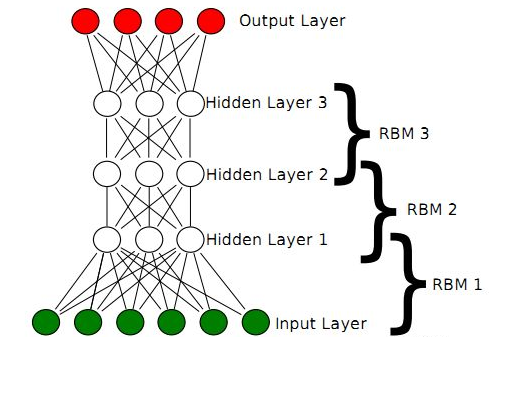回想一下RBM，由可见层、隐层组成，可见单元用于接受输入，隐含单元用于提取特征，因此隐含单元也有个别名，叫特征检测器。也就是说，通过RBM训练之后，可以得到输入数据的特征。
另外，RBM还通过学习将数据表示成概率模型，一旦模型通过无监督学习被训练或收敛到一个稳定的状态，它还可以被用于生成新数据。

正是由于RBM的以上特点，使得DBN逐层进行训练变得有效，通过隐层提取特征使后面层次的训练数据更加有代表性，通过可生成新数据能解决样本量不足的问题。逐层的训练过程如下：
（1）最底部RBM以原始输入数据进行训练
（2）将底部RBM抽取的特征作为顶部RBM的输入继续训练
（3）重复这个过程训练以尽可能多的RBM层

Hinton提出，这种预训练过程是一种无监督的逐层预训练的通用技术，也就是说，不是只有RBM可以堆叠成一个深度网络，其它类型的网络也可以使用相同的方法来生成网络。

###### 参考文献

https://www.cnblogs.com/jhding/p/5687696.html https://blog.csdn.net/u010223750/article/details/60882390
https://blog.csdn.net/itplus/article/details/19408143
Hinton G. A practical guide to training restricted Boltzmann machines[J]. Momentum, 2010, 9(1): 926.
Bengio Y, Lamblin P, Popovici D, et al. Greedy layer-wise training of deep networks[J]. Advances in neural information processing systems, 2007, 19: 153.
Hinton G E, Osindero S, Teh Y W. A fast learning algorithm for deep belief nets[J]. Neural computation, 2006, 18(7): 1527-1554.
https://en.wikipedia.org/wiki/Restricted_Boltzmann_machine
https://blog.csdn.net/Rainbow0210/article/details/53010694?locationNum=1&fps=1
https://my.oschina.net/u/876354/blog/1626639

展开全文机器学习 深度学习 深度置信网络
• 本节终于来到了重头戏受限玻尔兹曼机，为了能深入理解本节，我们深入讲了很多基础知识，这些基础知识很重要，是理解本节的基础，同时也是你学习其他算法的基础如强化学习、自然语言处理等。本节的安排是先对比一下...
• ... 尽管性能没有流行的生成模型好，但受限玻尔兹曼机还是很多读者都希望了解的内容。这不仅是因为深度学习的复兴很大程度上是以它为前锋，同时它那种逐层训练与重构的思想也非常有意思。...
• 首先采用多层无监督卷积受限玻尔兹曼机自动对.肺结节图像进行特征学习，然后利用分类受限玻尔兹曼机对获得的特征进行良恶性分类。为避免分类受限玻.尔兹曼机在训练中出现的特征同质化问题，引入了交叉熵稀疏惩罚对其...研究论文
• 最近在项目中接触到了一些判别受限玻尔兹曼机DRBM的应用，于是就深入学习了一下它的历史进程，受限玻尔兹曼机RBM到深度信念网络DBN，还有深度玻尔兹曼机DBM等等到底是啥。深度信念网络DBN
• ## 干货 | 受限玻尔兹曼机基础教程

千次阅读 热门讨论 2018-03-28 00:00:00
定义与结构受限玻尔兹曼机（RBM）由Geoff Hinton发明，是一种用于降维、分类、回归、协同过滤、特征学习和主题建模的算法。下载pdf，请后台回复关键词：20180328目录：1. 定义与结构2. 重构3. 概率分布4. 多层结构5....
• 受限玻尔兹曼机(Restricted Boltzmann Machine,简称RBM)是由Hinton和Sejnowski于1986年提出的一种生成式随机神经网络(generative stochastic neural network)，该网络由一些可见单元(visible unit，对应深度学习 机器学习 神经网络
• 受限玻尔兹曼机 该系列的其他文章： 常见的五种神经网络(1)-前馈神经网络 常见的五种神经网络(2)-卷积神经网络 常见的五种神经网络(3)-循环神经网络(上篇) 常见的五种神经网络(3)-循环神经网络(中篇) 常见的五种...深度学习 神经网络
• RBM被认为是受限的，因为没有两...玻尔兹曼机是一种随机神经网络，借鉴了模拟退火思想。 玻尔兹曼机的网络模型与BP神经网络的结构没有什么区别，只是训练的方式不太一样 玻尔兹曼机简单地将就是一个无向图模型 ...
•   就像普通的玻尔兹曼机，受限玻尔兹曼机也是基于能量的模型，其联合概率分布由能量函数指定（能量函数的概念最早来自于统计热力学家研究磁体的易辛模型，后来被Hinton借鉴发展为RBM模型） Pθ(v=v,h=h)=1Zexp(−...
• 受限玻尔兹曼机起源于图模型的神经网络。这种神经网络是由Hopfield\text{Hopfield}Hopfield神经网络那样的相互连接型网络衍生而来的。本部分首先介绍Hopfield\text{Hopfield}Hopfield神经网络和玻尔兹曼机，然后介绍...神经网络 深度学习
• 受限玻尔兹曼机（RBM） 1、玻尔兹曼分布  玻尔兹曼分布是统计物理中的一种概率分布，描述系统处于某种状态的概率     2、网络结构  可见单元-输入数据  隐藏单元-计算得到的结果  二部图-图的节点集合...
• 然而，有一种更好的神经网络模型，这就是受限玻尔兹曼机。使用层叠波尔兹曼机组成深度神经网络的方法，在深度学习里被称作深度信念网络DBN，这是目前非常流行的方法。下面的术语，将把自联想网络称作自编码网络...
• 最后介招由多个受限玻尔兹曼机推叠组成的深度信念网络 4.1 Hopfield神经网络 前几章介绍过的多层神经网络, 如图4.1所示的相互连接型 相互连接型网络不分层,单元之间相互连接。 能根据单元的值记忆网络状态,...
• 受限玻尔兹曼机（RBM）由Geoff Hinton发明，是一种用于降维、分类、回归、协同过滤、特征学习和主题建模的算法。（如需通过实例了解RBM等神经网络的应用方法，请参阅应用案例)。 我们首先介绍受限玻尔兹曼机这类...
• 尽管性能没有流行的生成模型好，但受限玻尔兹曼机还是很多读者都希望了解的内容。这不仅是因为深度学习的复兴很大程度上是以它为前锋，同时它那种逐层训练与重构的思想也非常有意思。本文介绍了什么是受限玻尔兹曼机...
• 感知的模型大家都比较熟悉，它是一个有若干输入和一个输出的模型，如下图: 输出和输入之间学习到一个线性关系，得到中间输出结果： 接着是一个神经元激活函数: 从而得到我们想要的输出结果1或者-1。 这个...
• 3、DBN训练方法： DBN 是由多层 RBM 组成的一个神经网络，它既可以被看作一个生成模型，也可以当作判别模型，其训练过程是：使用非监督贪婪逐层方法去预训练获得权值。 训练DBN的过程是一层一层地进行的。其中，上...
• DeepLearning 0.1 documentation中文翻译: Restricted Boltzmann Machines (RBM)_受限玻尔兹曼机 原文网址: http://deeplearning.net/tutorial/rbm.html DeepLearning 01 documentation中文翻译 Restricted ...
• 接着分别讲述了：(1) 其基本组成结构——受限玻尔兹曼机的的基本情况，以及，(2) 这个基本结构如何组成深度信念网络。 本文仅仅能使读者了解深度信念网络这一概念，内容非常浅显，甚至有许多不严密的地方。如果有...
• ## 玻尔兹曼机

千次阅读 2021-02-21 09:45:48
受限玻尔兹曼机RBM： 如图RBM是一个二层结构： 上层表示 n 个隐藏单元构成的隐藏(输出)层，一般服从伯努利分布； 下层表示m个可视单元构成的可视(输入)层，一般服从高斯或伯努利分布； RBM有两层，隐藏层、...神经网络 深度学习 机器学习...